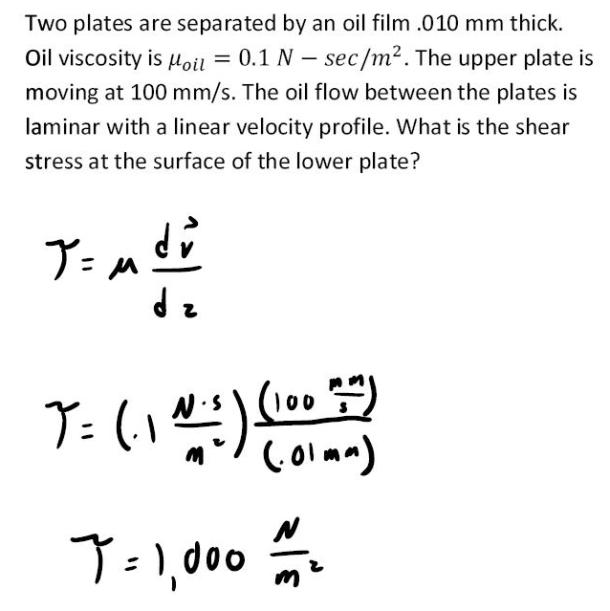fluid mechanics math problems engineering equations fluid mechanics basic mechanics school homework engineering math fluids formulas fluid mechanics problems fluid mechanics problem solutions to fluids problems full solution fluid mechanics engineering fluids problem solution fluid mechanics math problems engineering equations fluid mechanics basic mechanics school homework engineering math fluid mechanics formulas fluid problems fluid mechanics problem solutions to fluid mechanics problems full solution fluid mechanics engineering fluid mechanics problem solution
fluid mechanics math problems engineering equations fluid mechanics basic mechanics school homework engineering math fluids formulas fluid mechanics problems fluid mechanics problem solutions to fluids problems full solution fluid mechanics engineering fluids problem solution fluid mechanics math problems engineering equations fluid mechanics basic mechanics school homework engineering math fluid mechanics formulas fluid problems fluid mechanics problem solutions to fluid mechanics problems full solution fluid mechanics engineering fluid mechanics problem solution
Highalphabet Home Page fluid mechanics solutions fluids math solved Fluid Mechanics Page 1
Two plates are separated by an oil film .01 mm thick. Oil viscosity is .1 N-sec/m^2. the upper plate is moving at 100 mm/s. The oil flow between the plates is laminar with a linear velocity profile. What is the shear stress at the surface of the lower plate?Two plates are separated by an oil film .01 mm thick. Oil viscosity is .1 N-sec/m^2. the upper plate is moving at 100 mm/s. The oil flow between the plates is laminar with a linear velocity profile. What is the shear stress at the surface of the lower plate?